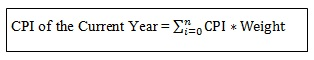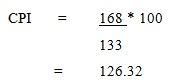# How to Calculate Consumer Price Index (CPI)

Calculating Consumer Price Index (CPI) involves measuring changes in price levels of a sample of representative goods and services used by the households in an economy over a specific period. This market basket of commodities is developed based on the information provided by the households regarding their expenditures on different product and service categories. Calculation of CPI takes two types; CPI for single item and for multiple item. How to calculate the Consumer Price Index (CPI) for single and for multiple item is explained here in detail.

## Formula to Calculate CPI for a single item

CPI for a single item can be calculated using following formula

##Fomula to Calculate CPI for multiple items

This is an approach which uses weighted averages of the indices and sub-indices that weights amount 1 or 100. That means, in order to simplify the calculation making an allowance for prices of thousands of products and services, each price is given with a specific weight . The weighting is determined based on the importance of the quantity of products and services that are being consumed. CPI for multiple items can be calculated using following formula.Graphical representation of the changes in CPI constructed over a period compared to the base year is illustrated by the consumer price index chart. This chart enables the reader to have an understanding about the minimum and maximum changes of CPI over a given period, mean and median of CPIs as well as substantial deviations of CPIs compared to the base year.

## Four steps to calculate consumer price index (CPI)

CPI is constructed through four main steps.

Step 01– A base year is selected for the calculation. The CPI of the base year is set as 100.

Step 02 – Based on how a typical consumer spends his / her money on purchasing commodities, a basket of goods and services is defined for the base year. In order to gather this information, the national body of authority conducts several surveys with consumers and households. Then prices of each of those products is added together in the base year to arrive at the price of base year.

Step 03 – Prices of the same commodity basket at the current year is added together as the third step.

Step 04 – Calculate the CPI using the CPI formula. This includes dividing the current year prices from the prices of base year and multiplies that by the CPI of the base year which is 100.

Following example illustrates this process in a meaningful manner.

## Calculate consumer price index (CPI) – Example

Assume that the market basket of goods and services of a given economy is as mentioned below for two given time periods.Consider the base year as 2000.

Based on the above information, CPI can be calculated as follows.This answer implies that the prices of the basket of goods and services have been increased by 26.32% from 2000 to 2010.